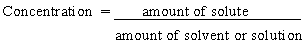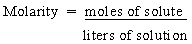## Solutions

 Solute, Solvent, and Solution Examples of Solutions Calculations Based on Percent By Mass Data Concentration Molarity Dilution Titration

Solute, Solvent, and Solution

It is important to distinguish between three closely related terms solute, solvent, and solution.

 Solute – The substance that dissolves to form a solution Solvent – The substance in which a solute dissolves Solution – A mixture of one or more solutes dissolved in a solvent

The following rules can be used to decide which component of a solution is the solute and which is the solvent.

• There are three states of matter: solids, liquids, and gases. Any reagent that undergoes a change in state when it forms a solution is the solute.
• If neither reagent changes state, the reagent present in the smallest amount is the solute.

The table below gives examples of different kinds of solutions. We normally think of solutions as the result of dissolving a solid such as NaCl in a solvent such as water. (NaCl is the solute in this solutions because it changes state when it dissolves.)

It is also possible to dissolve gases such as HCl in a liquid to form a solution such as hydrochloric acid. It is even possible to dissolve gases in solids, such as H2 in platinum metal. Or liquids in solids, such as mercury in sodium to form an alloy or amalgam of the two metals. Wine that is 12% alcohol by volume is a solution of a small quantity of alcohol (the solute) in a larger volume of water (the solvent).

 Practice Problem 10:Use the density of mercury (13.60 g/cm3) to calculate the number of atoms in a liter of this liquid. Click here to check your answer to Practice Problem 10 Click here to see a solution to Practice Problem 10Examples of Solutions

 Solute Solvent Solution NaCl(s) H2O(l) NaClaq) H2(g) Pt(s) H2/Pt(s) Hg(l) Na(s) Na/Hg(s) alcohol water wineCalculations Based on Percent-by-Mass Data

Chemists have historically described solutions in terms of percent-by-mass of one or more components of a mixture. By combining this information with measurements of the density of the solution we can determine the number of moles of each component.

 Practice Problem 11:Concentrated sulfuric acid is 96.0% H2SO4 by mass. (The remaining 4% is water.) The density of this solution is 1.84 g/cm3. Calculate the number of moles of H2SO4 in a liter of concentrated sulfuric acid. Click here to check your answer to Practice Problem 11 Click here to see a solution to Practice Problem 11Extensive quantities such as mass and volume are often used when preparing solutions. We can talk about adding a teaspoon of sugar when we make ice tea, for example, or filling a 2-L pitcher with ice tea. But when we want to compare solutions, we need intensive quantities that tell us how much sugar has been added to a given volume of lemonade or ice tea. We need to know the concentration of the solution, which is the ratio of the amount of solute to the amount of either solvent or solution.There are many different ways in which the concentration of a solution can be reported, but concentration units always describe the ratio of the amount of solute in a sample to the amount of either the solvent or the solution.Because so many calculations are based on the concept of the mole, the most commonly used concentration unit is based on the number of moles of solute per liter of solution.Practice Problem 12:What is the concentration of the Cu2+ ion in a solution prepared by dissolving 1.25 grams of CuSO4 · 5 H2O in enough water to give 50.0 mL of solution? Click here to check your answer to Practice Problem 12 Click here to see a solution to Practice Problem 12

 Practice Problem 13:Calculate the volume of 1.50 M HCl that would react with 25.0 grams of CaCO3 according to the following balanced equation. CaCO3(s) + 2 HCl(aq)Ca2+(aq) + 2 Cl-(aq) + CO2(g) + H2O(l) Click here to check your answer to Practice Problem 13 Click here to see a solution to Practice Problem 13

 Practice Problem 14:Assume that a metal reacts with hydrochloric acid according to the following balanced equation. M(s) + 2 HCl(aq)M2+(aq) + 2 Cl-(aq) + H2(g) Calculate the atomic weight of the metal if 125 mL of 0.200 M HCl reacts with 0.304 g of the metal. M + 2 HClMCl2 + H2 Click here to check your answer to Practice Problem 14 Click here to see a solution to Practice Problem 14Dilution

Adding more solvent to a solution to decrease the concentration is known as dilution. Starting with a known volume of a solution of known molarity, we should be able to prepare a more dilute solution of any desired concentration.

 Practice Problem 15:Describe how you would prepare 2.50 liters of an 0.360 M solution of sulfuric acid starting with concentrated sulfuric acid that is 18.0 M. Click here to check your answer to Practice Problem 15 Click here to see a solution to Practice Problem 15Titration

We can measure the concentration of a solution by a technique known as a titration. The solution being studied is slowly added to a known quantity of a reagent with which it reacts until we observe something that tells us that exactly equivalent numbers of moles of the reagents are present. Titrations are therefore dependent on the existence of a class of compounds known as indicators.

The endpoint of an acid-base titration is the point at which the indicator turns color. The equivalence point is the point at which exactly enough base has been added to neutralize the acid.

 Practice Problem 16:The reaction between oxalic acid (H2C2O4) and sodium hydroxide (NaOH) can be described by the following equation. H2C2O4(aq) + 2 NaOH(aq)2 Na+(aq) + C2O42-(aq) + 2 H2O(l) Calculate the concentration of an oxalic acid solution if it takes 34.0 mL of an 0.200 M NaOH solution to consume the acid in a 25.0 mL sample of this oxalic acid solution. Click here to check your answer to Practice Problem 16 Click here to see a solution to Practice Problem 16